yicaoting
★

NanKing, China,
2011-11-08 14:40
(3633 d 01:41 ago)

(edited by yicaoting on 2011-11-08 15:21)
Posting: # 7636
Views: 9,936

## The secret of WinNonlin's BE's post-hoc Power analysis [Software]

Dear all,

As asked by HS and provoked by this, it's time to talk about WNL's BE's post-hoc Power analysis.

For a long time, WNL's Power is considered as statistical NONSENSE, see:
HS's presentations such as

Of course, I agree with our HS's opinion on this issue largely because of the strange method used by WNL for Power calculation, and the strange results as well.

Actually, I have consulted a medical statistician who is professional at design and analysis of clinical trials. He told me he usually do power analysis to determine sample size before starting a trial, but seldom calc post-hoc power, he also told me that even if the post-hoc power is a little lower than designed or expected power, it is no necessary to do additional trial to increase the sample size and expect to increase the power.
Consistent with HS’s and Potvin's opinions.

Regardless of the necessity, let's first look inside into WNL to see how it calc power.

----------From PNX WNL User Guide P341-342----------------------------------
Power
Power is the post-hoc probability of detecting a difference greater than or equal to a specified percentage of the reference least squares mean. In general,

Power = 1 – (probability of a type II error)
= probability of rejecting H0 when H1 is true.

In the bioequivalence calculations, the hypotheses being tested are:

H0: RefLSM = TestLSM
H1: RefLSM ≠ TestLSM

For the no-transform case, the power is the probability of rejecting H0 given:

|TestLSM – RefLSM | ≥ fractionToDetect × RefLSM

For ln-transform, and data already ln-transformed, this changes to:

|TestLSM – RefLSM | ≥ – ln(1 – fractionToDetect),

and similarly for log10-transform and data already log10-transformed.

For the sake of illustration, assume fractionToDetect = 0.2, RefLSM>0, and no transform was done on the data. Also the maximum probability of rejecting H0 occurs in the case of equality,
|TestLSM – RefLSM| = 0.2×RefLSM. So:

Power = Pr (rejecting H0 at the alpha level given |Difference| = 0.2×RefLSM)
= Pr (|Difference/DiffSE| > t(1-α/2),df given |Difference| = 0.2×RefLSM)
= Pr (Difference/DiffSE > t(1-α/2),df given |Difference| = 0.2×RefLSM)
+ Pr (Difference/DiffSE < –t(1-α/2),df given |Difference| = 0.2×RefLSM)

Let:

t1 = t(1-α/2),df – 0.2×RefLSM / DiffSE
t2 = – t(1-α/2),df – 0.2×RefLSM / DiffSE
tstat = (Difference – 0.2×RefLSM) / DiffSE

Then:

Power = Pr(tstat > t1 given |Difference| = 0.2×RefLSM)
+ Pr(tstat < t2 given |Difference| = 0.2×RefLSM)

Note that tstat is T-distributed. Let p1 be the p-value associated with t1 (the area in the tail), and let p2 be the p-value associated with t2 (the area in the tail; note that p2 may be negligible). Then:

Power = 1 – (p1 – p2)

----------From PNX WNL User Guide P341-342----------------------------------
regretfully, WNL doesn't give us the formula when data is ln=transformed before BE analysis.

Before we start to validate WNL's formula, let me show my first puzzle:
As pointed by above WNL's user guide, it is obviouse that WNL is calculating the Power for TOST, but in WNL's ASCII output WNL gives us
 Power of ANOVA for Confidence Level 90.00     Power at 20% = 0.993541 
for ANOVA or for TOST? It is a question.

Now, let me validate WNL's formula using Chow and Liu's dataset.

-----For original data without transformation--------
WNL's result is
 Power of ANOVA for Confidence Level 90.00        Power at 20% = 0.9935408 

My formula and result is:
t1 = t (1-α/2),df – (1 - 0.8)×RefLSM / DiffSE t2 = – t (1-α/2),df – (1.2 -1)×RefLSM / DiffSE p1 = TDist(Abs(t1), n1 + n2 - 2, 1) p2 = TDist(Abs(t2), n1 + n2 - 2, 1) Power = 1 - (p1 - p2)

Power Analysis -------------------------------------------------------------------------- Parameter  T stat 1     T stat 2     P value 1     P value 2     Power -------------------------------------------------------------------------- AUC      -2.705765713 -6.140054462  0.006455207   0.000001760  0.993546553 --------------------------------------------------------------------------

------------For ln-transformed data---------------
WNL's result is
  Power of ANOVA for Confidence Level 90.00         Power at 20% = 0.9839865

My formula and result is:
t1 = t (1-α/2),df + Ln(0.8) / DiffSE t2 = – t (1-α/2),df - Ln(1.25) / DiffSE p1 = TDist(Abs(t1), n1 + n2 - 2, 1) p2 = TDist(Abs(t2), n1 + n2 - 2, 1) Power = 1 - (p1 - p2)

Power Analysis -------------------------------------------------------------------------- Parameter  T stat 1      T stat 2     P value 1     P value 2     Power -------------------------------------------------------------------------- ln(AUC)  -2.289520539  -5.723809288  0.016004731   4.65843E-06   0.983999927 --------------------------------------------------------------------------

To ensure the sufficient precision of my calc, all the above calculations were done using MS Excel 2010 which is of much more higher precision than MS Excel 2000, 2003 and 2007 when Tinv() or Tdist() is used.
For comparison:
   Software                Tinv(0.1,22) R 2.10.1                1.717144374380243 Excel 2010              1.71714437438024 Open Office 3.3.0       1.71714437438025 Gnumeric 1.10.16        1.71714437438148 Excel 2003 and 2007     1.71714433543983 WNL 5.1.1               1.71714434835526

It should be noted that the formula I used for Ln-transformed data is of no reference, only obtained by my guess, but it works well specially to obtain WNL's results. I don't know whether it is correct or not and don't know WNL is correct or not.

If we using PowerTOST as a gold standard for power calc of TOST, all WNL's results areand totally wrong.

It seems that WNL's post-hoc power calc doesn't directly use Observed Diff or Observed Ratio or intra-CV, only indirectly uses DiffSE and Expected Diff or Ratio such as 0.8, 1,2, 0.8, 1.25. Dear all, True or False of WNL's method?

Dear HS, have you reached Berlin and meet our D. Labes? I need your insight and comments on this issue.
yicaoting
★

NanKing, China,
2011-11-08 14:55
(3633 d 01:26 ago)

(edited by yicaoting on 2011-11-08 15:11)
@ yicaoting
Posting: # 7637
Views: 7,972

## The secret of WinNonlin's BE's post-hoc Power analysis

go on validating WNL's and my formula using unbalanced data

Chow and Liu's famous data, delete Subject # 23 and 24's data both in period 1 and period 2.

For original data-----------------
WNL's result is     Power of ANOVA for Confidence Level 90.00     Power at 20% = 0.99266427

My result is
Power Analysis    ----------------------------------------------------------------------------- Parameter   T stat 1     T stat 2     P value 1     P value 2     Power ----------------------------------------------------------------------------- AUC       -2.671468902  -6.120905388  0.007332086  2.78018E-06   0.992670694 -----------------------------------------------------------------------------

For ln-transformed data----------
WNL's result is
    Power of ANOVA for Confidence Level 90.00     Power at 20% = 0.97882724

My result is
Power Analysis    ----------------------------------------------------------------------------- Parameter   T stat 1     T stat 2     P value 1     P value 2     Power ----------------------------------------------------------------------------- ln(AUC)   -2.168810573  -5.618247059  0.02116397   8.44382E-06   0.978844474 -----------------------------------------------------------------------------

also same at level of 0.0001. Seems my formula works as WNL's inside hiden formula.

The level of the precision is interestingly same to that of BE's 90% CI.

go on validating WNL's and my formula using full data

Original data, use 93% CI, 20% diff to detect WNL's power = 0.99020906 My power    = 0.990218894 Original data, use 93% CI, 13% diff to detect WNL's power = 0.82872712 My power    = 0.828839088 Ln-transed data, use 93% CI, 20% diff to detect WNL's power = 0.97637515 My power    = 0.976397234 Ln-transed data, use 93% CI, 13% diff to detect WNL's power = 0.72133308 My power    = 0.721483927

All the results are satisfactory. Seems I have touched into WNL's black box.

Edit: Merged two posts.
Helmut
★★★Vienna, Austria,
2011-11-08 15:06
(3633 d 01:15 ago)

@ yicaoting
Posting: # 7638
Views: 8,035

## The secret of WinNonlin's BE's post-hoc Power analysis

Dear yicaoting,

thanks for stepping into murky waters.

» It seems that WNL's post-hoc power calc doesn't directly use Observed Diff or Observed Ratio or intra-CV, only indirectly uses DiffSE and Expected Diff or Ratio such as 0.8, 1,2, 0.8, 1.25. Dear all, True or False of WNL's method?

Fascinating. I’m a little bit busy now, but will have a look as soon as possible.

» Dear HS, have you reached Berlin and meet our D. Labes?
1. Yes.
2. Tomorrow afternoon.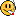Dif-tor heh smusma 🖖
Helmut SchützThe quality of responses received is directly proportional to the quality of the question asked. 🚮
Science Quotes
d_labes
★★★

Berlin, Germany,
2011-11-10 09:49
(3631 d 06:32 ago)

@ yicaoting
Posting: # 7646
Views: 7,878

## Power of superiority

Dear yicaoting, dear all,

» ----------From PNX WNL User Guide P341-342----------------------------------Power
» ...
» In the bioequivalence calculations, the hypotheses being tested are:
»
» H0: RefLSM = TestLSM
» H1: RefLSM ≠ TestLSM
» ...

This explains all! These hypotheses are for a superiority test or test for difference! Thus the first sentence should correctly read "In the bioequivalence calculations, the hypotheses not being tested ..."In BE testing we are interested in testing the reversed hypotheses, i.e. the Null is inequivalence, the Alternative is equivalence RefLSM = TestLSM (within some reasonable chosen margins).

This explains why the power given in WLN is higher then calculated by PowerTOST. It's well known that the superiority test has higher power than the equivalence or non-inferiority test.

The formulas given by yicaoting point in the same direction (power for a test of difference), but complicated by the fact that not the usual non-central t-distribution is used in the power calculation but an approximation via 'shifted' central t distribution.
I can't reproduce the numbers because I don't have RefLSM and the DiffSE. Yicaoting, be so kind to post them here.

» Dear all, True or False of WNL's method?
I would state: Approximate correct for the problem stated but answers a question no one has asked.

Regards,

Detlew
Helmut
★★★Vienna, Austria,
2011-11-10 14:39
(3631 d 01:42 ago)

@ d_labes
Posting: # 7650
Views: 7,867

## Power of superiority

Dear Detlew,

was really nice meeting you yesterday!
BTW, fog at both Berlin’s and Vienna’s airports; arrived at home at midnight…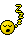» I can't reproduce the numbers because I don't have RefLSM and the DiffSE. Yicaoting, be so kind to post them here.

Results form PHX/WNL’s BE output (standard model; subject(sequence) random):

Full dataset (balanced; nRT=nTR=12)
Untransformed
LSMRef   82.559375          SERef  4.34005723555842
LSMTest  80.271875          SETest 4.34005723555842
LSMDiff  -2.28750000000002  SEDiff 3.73326038107666
‘Power’   0.9935408
ln transformed
LSMRef   4.37972882180257   SERef  0.05625992661764
LSMTest  4.35107672956901   SETest 0.05625992661764
LSMDiff -0.028652092233559  SEDiff 0.055693090418743 (corrected; see here – was 0.02865…)
‘Power’  0.9839865

Reduced dataset (imbalanced; 23/24 excluded: nRT=10, nTR=12)
Untransformed
LSMRef   83.9116666666666   SERef  4.59672942436518
LSMTest  81.5147916666666   SETest 4.59672942436518
LSMDiff  -2.39687499999999  SEDiff 3.81747472994938
‘Power’   0.99266427
ln transformed
LSMRef   4.39812486326424   SERef  0.059448124239987
LSMTest  4.35107672956901   SETest 0.059448124239987
LSMDiff -0.032751848318541  SEDiff 0.057311390736542
‘Power’  0.97882724

» I would state: Approximate correct for the problem stated but answers a question no one has asked.

Great.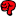Dif-tor heh smusma 🖖
Helmut SchützThe quality of responses received is directly proportional to the quality of the question asked. 🚮
Science Quotes
d_labes
★★★

Berlin, Germany,
2011-11-10 16:42
(3630 d 23:39 ago)

@ Helmut
Posting: # 7652
Views: 7,929

## Power of equality

Dear Helmut,

» was really nice meeting you yesterday!

Meeting You, yes it was, really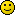!
» BTW, fog at both Berlin’s and Vienna’s airports; arrived at home at midnight…For that I feel sorry for you. Eventually we should had smoked to a lesser degree?Regarding the WLN power I must correct myself to a certain degree.
Chow, Shao and Wang1) call a test with the hypotheses pair   H0 µT = µR  HA µT ≠ µR 
a test of equality to distinguish it from a superiority test with the hypothesis pair   H0 µT-µR ≤ delta  HA µT-µR > delta
with delta the superiority margin. Ok, I think this is semantics.

These authors give for the cross-over design the following formula for the power of the 'equality test' (have translated it in R notation):
  power = 1 - pt(tcrit,df,ncp=eps*sqrt(2*n)/sigma)             + pt(-tcrit,df,ncp=eps*sqrt(2*n)/sigma)
where eps is the true difference for which the power shall calculated and tcrit is the quantile of the central t-distribution to the confidence level 1-alpha/2 with df = n-2 degrees of freedom.
Setting eps=0.2*LSMref and sigma/sqrt(2*n)=SEdiff and let  b=0.2*LSMref/SEdiff
we get    power = 1 - pt(tcrit,df,ncp=b) + pt(-tcrit,df,ncp=b)
With the approximation of the non-central t-distri via 'shifted' central t-distri according to    pt(x,df,ncp) ~ pt(x-ncp,df)
we obtain yicaotings formulas!

Lets calculate via non-central t-distribution using your given data:
Full dataset, untransformed
b  = 4.42291 df = 22 tcrit = qt(0.95,22) = 1.717144... power = 1 - 0.004184843 + 1.415275e-09 = 0.9958152
Seems to agree within the approximation used in WNL. At least the right order of magnitude.

Full dataset, logtransformed
b  = 30.57179 df = 22 tcrit = qt(0.95,22) = 1.717144... power = 1-2.395435e-180+0 = 1
Seem not to function! No agreement to the WNL results. Due to insufficient degree of approximation for large non-centrality or due to false formula?

b  = ln(0.8)/SEdiff = -7.788037 (yicaotings formula) df = 22 tcrit = qt(0.95,22) = 1.717144... power = 1-1+1 = 1
Seems also not to function! This is surprising!yicaoting: Which SEdiff did you use?

Reduced dataset is the homework for all.
Sorry for all that numbers with insufficient decimals.

1) Chow, Shao and Wang
"Sample size calculations in clinical research"
Marcel Dekker, New York, NY 2003

Regards,

Detlew
Helmut
★★★Vienna, Austria,
2011-11-10 17:00
(3630 d 23:21 ago)

@ d_labes
Posting: # 7653
Views: 7,846

## Power of equality

Dear Detlew,
» » BTW, fog at both Berlin’s and Vienna’s airports; arrived at home at midnight…» For that I feel sorry for you. Eventually we should had smoked to a lesser degree?Right. Maybe some cloud condensation nuclei leaked out… Vienna is smoker’s territory anyhow and the airport is just 1.8 km from river Danube. Was a starry night when I arrived home.» Chow, Shao and Wang…
» […] I think this is semantics.

Me too.

» Reduced dataset is the homework for all.

Yes, sir. ASAP.

Dif-tor heh smusma 🖖
Helmut SchützThe quality of responses received is directly proportional to the quality of the question asked. 🚮
Science Quotes
d_labes
★★★

Berlin, Germany,
2011-11-11 09:55
(3630 d 06:26 ago)

@ Helmut
Posting: # 7656
Views: 8,147

## Power of equality test - 2nd try for the logs

Dear Helmut,

seems there is an error / copy and paste mistake in your SEdiff of the full set, log-transformed.
My beasty number cruncher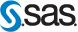(Proc MIXED) gives:

                              Least Squares Means Effect       Formulation  Estimate     Error    DF  t Value  Pr > |t|     Formulation  Referenc       4.3797   0.05626    22    77.85    <.0001  Formulation  Test           4.3511   0.05626    22    77.34    <.0001                        Differences of Least Squares Means                                                      Standard   Effect       Formulation  _Formulation  Estimate      Error     DF   t Value   Formulation  Referenc     Test           0.02865    0.05569     22      0.51 

So repeating the power calculations for the Full set, log-transformed :
b  = ln(0.8)/SEdiff = -4.006887 (yicaotings formula) df = 22 tcrit = qt(0.95,22) = 1.717144... power = 1 - 1 + 0.9872805 = 0.9872805
Seems reasonable in accordance to WNL I think.

So mystery resolved. Thanx to yicaoting.

Regards,

Detlew
Helmut
★★★Vienna, Austria,
2011-11-11 14:39
(3630 d 01:42 ago)

@ d_labes
Posting: # 7659
Views: 7,793

## Crtl-C/Ctrl-V

Dear Detlew!

» seems there is an error / copy and paste mistake in your SEdiff of the full set, log-transformed.

Oops! Clever enough to , too stupid to select the right column.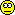» My beasty number cruncher(Proc MIXED) gives:
»                                                     Standard
»   Effect       Formulation  _Formulation Estimate      Error   DF  t Value
»   Formulation  Referenc     Test          0.02865    0.05569   22     0.51

PHX/WNL: LSMDiff -0.028652092233559  SEDiff 0.055693090418743

THX!

Dif-tor heh smusma 🖖
Helmut SchützThe quality of responses received is directly proportional to the quality of the question asked. 🚮
Science Quotes
yicaoting
★

NanKing, China,
2011-11-12 07:05
(3629 d 09:16 ago)

@ d_labes
Posting: # 7661
Views: 7,794

## Power of equality

Dear d_labes,

» yicaoting: Which SEdiff did you use?

My Diff and DiffSE are

Diff(T-R) = -0.0286520922335587
DiffSE = 0.055693090418743

the same to those of WNL.Ing. Helmut Schütz